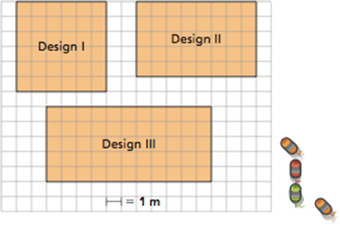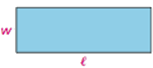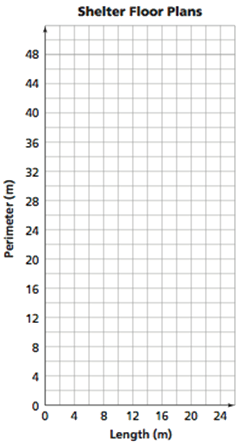Display Accessibility Tools

# CCSS.Math.Content.6.EE.A.1

Write and evaluate numerical expressions involving whole-number exponents.

## Covering and Surrounding Investigation 4

Exponential notation is originally introduced in Prime Time, and it reinforced throughout in Covering and Surrounding when developing area and volume formulas. For instance, in Problem 4.2B, students discuss whether the formula for volume of a rectangular prism, V=LWH, works for a cube.

### Problem 4.2 B

4. Dushane says that the formulas for Natasha's and Kurt's methods do not work for cubes because the volume of cube with side length l is l3. Do you agree with Dushane? Explain your reasoning.

# CCSS.Math.Content.6.EE.A.2.A

Write expressions that record operations with numbers and with letters standing for numbers. For example, express the calculation "Subtract y from 5" as 5 - y.

## Covering and Surrounding throughout the unit

Writing numeric and symbolic expressions and sentences to represent the operations required to solve problems is one of the most important skills in applied mathematics. Each unit of CMP3 includes many activities that develop understanding and proficiency in work on such modeling tasks.

Throughout Covering and Surrounding students develop and use formulas for perimeter and area of 2-dimensional shapes, and for surface area and volume of 3-dimensional shapes.

# CCSS.Math.Content.6.EE.A.2.B

Identify parts of an expression using mathematical terms (sum, term, product, factor, quotient, coefficient); view one or more parts of an expression as a single entity. For example, describe the expression 2 (8 + 7) as a product of two factors; view (8 + 7) as both a single entity and a sum of two terms.

## Covering and Surrounding throughout

The mathematical vocabulary of sum, product, factor and quotient are introduced in earlier units and used in Covering and Surrounding.  The mathematical term coefficient is not defined or used until problem 3.1 of Variables and Patterns. However, it is easily possible to introduce the term “coefficient” in Covering and Surrounding where formulas like P = 4s, and P = 2L + 2W, and A=(1/2) BH all involve coefficients.

Use of the word term to indicate any part of an algebraic or numeric expression consisting of a number, a letter, or the product of a number and a letter is not made explicit in any unit of the grade six CMP3 curriculum, except in the glossary of Variables and Patterns. We have avoided any attempt to define term because of the inherent ambiguity in the idea itself.  For example, does the expression 5(n + 7) involve two terms, three terms, or only one term?

The many CMP3 exercises involving the distributive property, like reasoning that perimeter of a rectangle can be expressed in two equivalent ways 2(L + W) and 2L + 2W highlight the need to view parts of complex expressions as single entities.  In the case of 2(L + W), order of operations conventions imply that one has to calculate the sum of length and width before multiplying that single number by 2.

# CCSS.Math.Content.6.EE.A.2.C

Evaluate expressions at specific values of their variables. Include expressions that arise from formulas used in real-world problems. Perform arithmetic operations, including those involving whole-number exponents, in the conventional order when there are no parentheses to specify a particular order (Order of Operations). For example, use the formulas V = s3 and A = 6 s2 to find the volume and surface area of a cube with sides of length s = 1/2.

## Covering and Surrounding throughout

Covering and Surrounding is the obvious place where students will develop skill in evaluating expressions for specific values, which occurs throughout the unit.

# CCSS.Math.Content.6.EE.A.3

Apply the properties of operations to generate equivalent expressions. For example, apply the distributive property to the expression 3 (2 + x) to produce the equivalent expression 6 + 3x; apply the distributive property to the expression 24x + 18y to produce the equivalent expression 6 (4x + 3y); apply properties of operations to y + y + y to produce the equivalent expression 3y.

## Covering and Surrounding Problem 1.1

The fundamental concept of equivalent expressions is a big idea throughout the CMP curriculum. The distributive property is first introduced in Prime Time, and threaded throughout the rest of the CMP3 units.  For instance, in Covering and Surrounding, Problem 1.1, the distributive property is used in a powerful way to explain equivalence of two common formulas of the perimeter of a rectangle:  P = 2L + 2W and P = 2(L+W).

C. Riverview School orders a bumper-car ride in the shape of a rectangle for their fundraising festival. The MARS company sends the school Designs I, II, and III.

1. What is the area of each design? Explain how you found the area.
2. What is the perimeter of each design? Explain how you found the perimeter.1. The dimensions of a rectangle called length l and width w. Look for patterns throughout Problem 1.1 to help you answer the questions below.1. Use words to describe a formula for finding the perimeter of a rectangle. Write the formula using symbols. Explain why it works.
2. Use words to describe a formula for finding the area of a rectangle. Write the formula using symbols. Explain why it works.
3. Find the perimeter and area of a rectangle with a width of 6 xwnrimwrwea NS lwnfrh od 15 centimeters.

# CCSS.Math.Content.6.EE.A.4

Identify when two expressions are equivalent (i.e., when the two expressions name the same number regardless of which value is substituted into them). For example, the expressions y + y + y and 3y are equivalent because they name the same number regardless of which number y stands for.

## Covering and Surrounding Problem 1.1

See above discussion of the distributive property and the equivalent forms for perimeter of a rectangle, P = 2L + 2W and P = 2(L+W).

# CCSS.Math.Content.6.EE.B.6

Use variables to represent numbers and write expressions when solving a real-world or mathematical problem; understand that a variable can represent an unknown number, or, depending on the purpose at hand, any number in a specified set.

## Covering and Surrounding Throughout

The use of letter variables to represent measurement values in the various geometric formulas occurs throughout Covering and Surrounding

# CCSS.Math.Content.6.EE.C.9

Use variables to represent two quantities in a real-world problem that change in relationship to one another; write an equation to express one quantity, thought of as the dependent variable, in terms of the other quantity, thought of as the independent variable. Analyze the relationship between the dependent and independent variables using graphs and tables, and relate these to the equation. For example, in a problem involving motion at constant speed, list and graph ordered pairs of distances and times, and write the equation d = 65t to represent the relationship between distance and time.

## Covering and Surrounding, Problems 1.2 and 1.3

For example, Covering and Surrounding problems 1.2 and 1.3 examine the relationship between perimeter and length for rectangles of fixed area and the relationship between area and length for rectangles of fixed perimeter.  In each case students are asked to express the relationship with a table and a graph of sample ordered pairs satisfying the relationship and then to describe the pattern relating values of the dependent variable to values of the independent variable (though the terms independent and dependent variable are not used at this point).

## Problem 1.2

The rangers in a national park want to build several storm shelters. The shelters must have 24 square meters of rectangular floor space.

A) Experiment with different rectangles that have whole-number dimensions. Sketch each possible floor plan on grid paper. Record your data in a table such as the one started below. Look for patterns, and describe the data.

Rectangle Length Width Perimeter Area
1 m x 24 m 1 m 24 m 50 m 24 m2

B) Suppose the walls are made of flat rectangular panels that are 1 meter wide and have the needed height.

1. What determines how many wall panels are needed, area or perimeter? Explain your reasoning.
2. Which design would require the most panels? Explain.
3. Which design would require the fewest panels? Explain.

### C)

1. Use your table to make a graph, such as the one below, to compare lengths and perimeters of various rectangles with an area of 24 square meters.2. Describe the shape of the graph. How do the patterns that you saw in your table show up in the graph?

### D)

1. Suppose you build a storm shelter with 36 square meters of rectangular floor space. Which design has the least perimeter? Which has the greatest perimeter? Explain your reasoning.
2. In general, describe the rectangle that has the greatest perimeter for a fixed, or unchanging, area. Describe the rectangle that has the least perimeter for a fixed area.

An extension of each given problem could also ask students to express the relationship as an equation with letter variable names.

For Problem 1.2, the equation for the constant area of 24 and changing perimeter is P = 2(X + 24/X).

Problem 1.3 is structured similarly, but it holds the perimeter constant and examines the changing area.For Problem 1.3, the equation for the constant perimeter and changing area is A = X (12 – X)